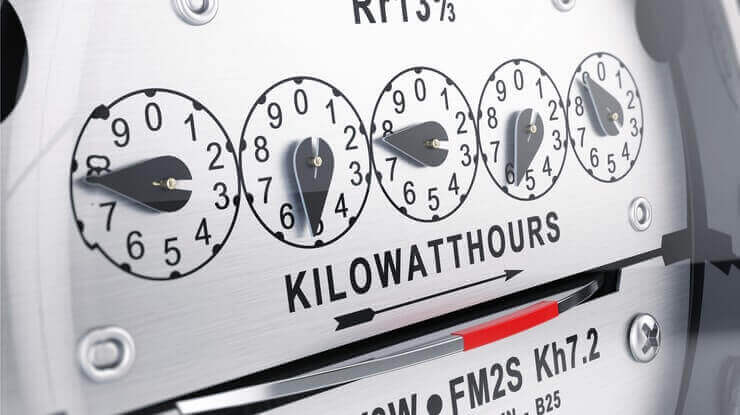# What Is A Kilowatt-hour (kWh) And What Can It Power?

Written By: Kelly Bedrich

A kilowatt-hour is a unit of measure for using one kilowatt of power for one hour. Just knowing what a kilowatt-hour is and what it can power can save you money on your electricity bill. Once you understand what is a kilowatt-hour, you can monitor electricity usage, make educated choices about saving energy, and lower your monthly electric bill.

## What is a Kilowatt-Hour?

A kilowatt-hour, otherwise known as a kWh, is a way to measure how much energy you’re using. It’s not the number of kilowatts you’re using in an hour, even though that seems to make sense.

A kWh equals the amount of energy you would use by keeping a 1,000 watt appliance running for one hour.

For instance, if you turned on a 100 watt bulb, it would take 10 hours to use one kilowatt-hour of energy. A 2,000 watt appliance, on the other hand, would only take half an hour. It all comes down to dividing the number of watts in an appliance into 1,000.

What is a Kilowatt-Hour?

A kilowatt-hour is a unit of measure for energy usage. It’s using one kilowatt of power (1000 watts) used for one hour. It’s abbreviated as kWh.

## Kilowatt-Hour vs. Kilowatt

What’s the difference between a kilowatt hour (kWh) and a kilowatt (KW)?

A kilowatt (kW) is 1,000 watts and is a measure of how much power something needs to run. In metric, 1,000 = kilo, so 1,000 watts equals a kilowatt.

A kilowatt hour (kWh) is a measure of the amount of energy something uses over time.

Think of it this way: a kilowatt (kW) is the amount of power something needs just to turn it on. A kilowatt hour (kWh) is the amount of power that device will use over the course of an hour.

Here’s an example: If you have a 1,000 watt drill, it takes 1,000 watts (or one kW) to make it work. If you run that drill for one hour, you’ll have used up one kilowatt of energy for that hour, or one kWh.

## What Can 1 Kilowatt-Hour Power?

Each item in your home will use a different amount of power. But if you want to know what 1 kWh can power, here are some examples:

• Running a dishwasher (1,000 watts): 1 hour
• Watching a 50″ LED TV (50 watts): 20 hours
• Cooking in the oven (2000 Watts): 30 minutes
• Running the refrigerator (300 Watts): 3 hours
• Using a Playstation 4 (150 Watts): 6.66 hours

How do I calculate what 1 kWh will power?

1. Locate the wattage for the device.
2. Convert the wattage from watts (W) to kilowatts (kW). To do that, just divide the number of watts by 1000.
3. Divide the number of kilowatts into 1kWh to see how long it takes for your device to use 1 kWh.

Here’s an example of how to calculate how long you can use an appliance with only 1 kWh of power.

How long can you play your Playstation 4 if you only use 1 kWh?

Number of Watts: 150 watts

150 watts / 1000 = .15 kilowatts

Number of Hours to use 1 kWh hour = 1 kWh / .15 kilowatts = 6.66 hours

Note that this doesn’t include the electricity used by the TV your game console may be connected to.

## How Many Kilowatt-Hours Do Common Household Items Use?

Obviously, every appliance in your home will use a different amount of power. And instead of looking at what you can do with a single kWh, it makes more sense to look at how much your appliances use under normal usage.

Here are examples of the number of kilowatt-hours common household items use:

• 50″ LED Television: around 0.016 kWh per hour
• Electric dishwashers: around 2 kWh per load
• Electric water heater: 380-500 kWh per month
• Refrigerator (24 cu. ft frost free Energy Star): 54 kWh per month
• Clothes Washer (warm wash, cold rinse): 2.3 kWh per load
• Clothes Dryer: 2.5 – 4.0 kWh per load
• Air Conditioner (3 ton 12 SEER): 3.0 kWh per hour
• Nissan Leaf Electric Car – 40 kWh per full battery charge

The Energy Guide label on newer appliances will include the estimated yearly electricity usage. Multiply that by your rate per kilowatt-hour and you have the cost to use that device.

## How Do I Calculate How Many kWh an Appliance Uses?

Your appliances account for around 25% of your electric bill. That includes your water heater, refrigerator, freezer and washer and dryer. You can easily calculate the number of kilowatt hours an appliance uses following these steps.

Time needed: 5 minutes

How to Calculate How Many Kilowatt Hours an Appliance Uses

1. Locate the wattage label on your appliance or electronic device.

Here are some examples of what you should look for.2. Write down the number of watts consumed by that appliance.

3. Write down how many hours each day you use the appliance.

4. Calculate the watt-hours per day

Multiply the number of watts consumed by the appliance by the number of hours per day you use the appliance. That calculates the total watt-hours you use per day

5. Calculate the kilowatt-hours per day.

The final step is to convert watt-hours into kilowatt hours. Divide your watt-hours per day by 1000 to get your kilowatt-hours per day.

Here’s an example of how to calculate the kWh for a refrigerator.

How many kWh does a refrigerator use?

Number of Watts: 300 watts

Hours used per day: 8 hours (while it keeps your food cold all day long, the motor is only running about 8 hours a day)

300 watts X 8 hours = 2,400 watt-hours per day

2,400 watt-hours per day / 1000 = 2.4 kWh per day

2.4 kWh per day X 30 days in a month = 72 kWh per month

Here’s an example of how to calculate the kWh for a dishwasher.

How many kWh does a dishwasher use?

Number of Watts:  1200 watts

Hours used per day:  2 hours

1200 watts X 2 hours = 2,400 watt-hours per day

2,400 watt-hours per day / 1000 = 2.4 kWh per day

2.4 kWh per day * 30 days in a month = 72 kWh per month

There are also several online resources and calculators that can help you with this, if you prefer that to pen, paper and calculator! Or create a spreadsheet to help you on this project.

Some consumers prefer to use smaller appliances in the kitchen, like a microwave oven, convection oven or toaster oven. Air fryers are a popular small kitchen appliance. Here’s an example of how much electricity an air fryer uses to cook fried chicken.

How Much Electricity Does an Air Fryer Use?

The average air fryer is 1450 watts. And the amount of electricity your air fryer uses will depend on what you are cooking and for how long.

Let’s say we’re cooking some air fryer fried chicken, which will take around 30 minutes at 400*.
Air Fryer Wattage: 1450
Time used: .5 hours
1450 watts X .5 hours = 725 watt-hours used
750 watt-hours / 1000 = .75 kWh to cook fried chicken in an air fryer.

So if you ate fried chicken every day for a month (yum), in a month your air fryer would use 22.5 kWh of electricity.

Additional Resources: What Appliances Use the Most Electricity

## How Many kWh Does a House Use Per Day?

One common question is, how many kWh does a house use per day? The amount of kWh you use will depend on:

• How big your residence is (square footage)
• The age of your home (which impacts your insulation, roofing materials and type of window)
• The number of people living there
• The type, number and age of appliances
• How you heat or cool your home
• Whether you have a pool
• The climate you live in

According to the EIA, in 2021, the average annual electricity consumption for a U.S. residential home customer was 10,632 kilowatt hours (kWh), an average of 886 kWh per month. That means the average household electricity consumption kWh per day is 29.5 kWh (886 kWh / 30 days).

Customers in some areas, like Texas, consume even more. The average annual household electricity consumption for a Texas home is 14,112 kWh. That’s 36% higher than the national average. Find out more about the average electricity bill in Texas with our article on that topic!

## Other Measures of Electricity: Amps, Ohms, Volts and Megawatts

Watts, kilowatts and kilowatt-hours are the measures of electricity you most commonly hear in US households. Here are some other common measures of electricity:

• Amp: an amp, short for ampere, is one unit of constant electrical current
• Volts: a volt (V) is a unit of electrical potential. Voltage is the potential for energy to move.
• Ohms: a measure of electrical resistance.
• Watts: a watt is a measure of power consumption.
What is a megawatt?

A megawatt (MW) is equal to one million watts. A megawatt-hours is a megawatt being used continuously for 1 hour. One megawatt hour will power approximately 330 homes for one hour.

What is a gigawatt?

A gigawatt (GW) is equal to one billion watts.